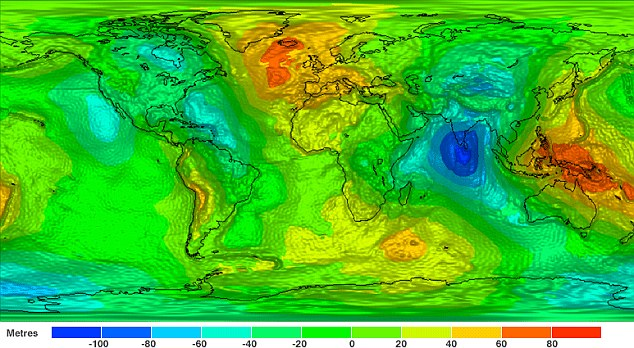In Physics by Brian Koberlein0 Comments

In introductory physics courses we often assume the force of gravity near the Earth is constant. That isn’t really true, since the farther you get from the Earth’s surface, the weaker its gravitational force becomes, but this change is very small. If you could stand on a platform 5 kilometers above the Earth, the gravitational force you feel would only be about 0.2% less than on the surface.

The rate at which the gravitational force varies with height is known as the gradient. By measuring the force of gravity at two different heights, we can determine the variation of the gravitational field. It is similar to the way you can measure the speed of a car by measuring its position at two different times. We’ve been able to measure gravitational gradients for some time, but a bigger challenge is measuring how the gradient changes with height, which is known as the curvature. Despite its name, “curvature” in this sense is not the same as the relativistic curvature of space. Now a team has successfully measured this curvature, and the results are published in Physical Review Letters.

To achieve this, the team used very cold clouds of atoms and tossed them in a vertical chamber. They then flashed the clouds with laser light from above and below. The first flash from above caused some of the atoms to absorb the light and become excited. These excited atoms also get a slight push from the laser pulse, so they shift relative to unexcited atoms in the cloud. The second pulse from below remixes the excited and unexcited atoms, which then interfere with each other quantum mechanically. The amount of interference depends upon how widely the two groups were separated, which depends upon the strength of the gravitational field around them. So by measuring the interference, the team could measure the acceleration of gravity to within a few millionths of a percent. The team did this measurement at three different heights, from which they could determine not only the gradient of gravity in this region, but also the curvature.

You might wonder why this is a big deal. After all, we know that gravity is an inverse square force, so a bit of math should tell us what the gradient and curvature is. While that’s true, it’s only true for “point” masses, or spherical masses of uniform density. The Earth is neither, so there are fluctuations of gravitational strength, gradient and curvature depending on where you are on Earth. Since these fluctuations depend upon the shape and density of Earth at different regions, this type of measurement useful for determine what lies underneath the Earth’s surface. The experiment could also be useful in better determining the universal constant of gravity.

In this particular work the team really performed a proof of concept. Rather than measuring the curvature of Earth’s gravity directly, they placed large weights around their device in order to give the region a stronger gravitational curvature. But with this experiment under their belt, the team can now work to increase the sensitivity to be useful for real-world applications.

Paper: G. Rosi, L. et al. Measurement of the Gravity-Field Curvature by Atom Interferometry. Phys. Rev. Lett. 114, 013001 (2015)